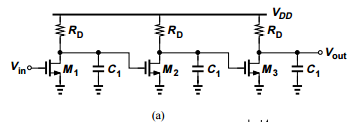+1-415-670-9189
info@expertsmind.com

Get Solution

Determine the condition for stability
Course:- Econometrics
Reference No.:- EM131099565

 TweetExpertsmind Rated 4.9 / 5 based on 47215 reviews.
Review Site
Assignment Help >> Econometrics

Unlike a one-pole system, the magnitude response of the circuit in Example 12.38 falls by more than 3 dB at the pole frequency.

Determine |H| at ωp. Can we say |H| falls 9 dB due to three coincident poles?

Example 12.38

We wish to apply negative feedback with K = 1 around the three-stage amplifier shown in Fig. 12.67(a). Neglecting other capacitances and assuming identical stages, plot the frequency response of the circuit and determine the condition for stability.

Assume λ = 0.Minimize

Ask Question & Get Answers from Experts
Browse some more (Econometrics) Materials
 What would have been the maximum possible difference between the interest rates on six-month lira and DM deposits? On three-month deposits? Do the answers surprise you? Give What is the amount of 10 equal annual deposits that can provide five annual withdrawals, where a first withdrawal of \$3,000 is made at the end of year 11 and subsequent with Now suppose that the demand increases by 10 units at each price.fill in the new quanity demanded in table above5.determine the new equilibrium price and quanity 6.reproduce th TLC Lawn Care, Inc. provides fertilizer and weed control services to residential customers. Its seasonal services package, regularly priced at \$250, includes several chemica There are H consumers and n firms. The demand of each consumer is x(p) = 1 - p and the cost function of each firm is c(y) = (y)^2/2. Compute the competitive equilibrium price, Consider the following version of the "chain store paradox". This entry game has the following timing: In the first stage an entrant decides whether or not to enter. In the Construct a table showing your total cost, average total cost, and marginalcost for output levels varying from 0 to 45 L. (HINT: There are 4 cups in alitre.) Draw the three Explain why the European Central Bank cannot selectively change interest rates in any of the 16 EU countries that have adopted the euro-for example, lowering the interest ra